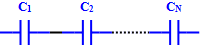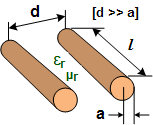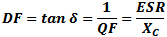About RF CafeCopyright: 1996 - 2024Webmaster:     BSEE - KB3UON RF Cafe began life in 1996 as "RF Tools" in an AOL screen name web space totaling 2 MB. Its primary purpose was to provide me with ready access to commonly needed formulas and reference material while performing my work as an RF system and circuit design engineer. The World Wide Web (Internet) was largely an unknown entity at the time and bandwidth was a scarce commodity. Dial-up modems blazed along at 14.4 kbps while tying up your telephone line, and a nice lady's voice announced "You've Got Mail" when a new message arrived... All trademarks, copyrights, patents, and other rights of ownership to images and text used on the RF Cafe website are hereby acknowledged. My Hobby Website: AirplanesAndRockets.com# Capacitors & Capacitance FormulasCapacitors are passive devices used in electronic circuits to store energy in the form of an electric field. They are the compliment of inductors, which store energy in the form of a magnetic field. An ideal capacitor is the equivalent of an open circuit (infinite ohms) for direct currents (DC), and presents an impedance (reactance) to alternating currents (AC) that depends on the frequency of the current (or voltage). The reactance (opposition to current flow) of a capacitor is inversely proportional to the frequency of the of the signal acting on it. Capacitors were originally referred to as "condensers" for a reason that goes back to the days of the Leyden Jar where electric charges were thought to accumulate on plates through a condensation process.

The property of capacitance that opposes a change in voltage is exploited for the purpose of conducting signals with a higher frequency component while preventing signals of lower frequency components from passing. A common application of a capacitor in an RF (radio frequency) circuit is where there is a DC bias voltage that needs to be blocked from being present in a circuit while allowing the RF signal to pass. DC power supplies use large capacitance values in parallel with the output terminals to smooth out low frequency ripples due to rectification and/or switching waveforms.When used in series (left drawing) or parallel (right drawing) with its circuit compliment, an inductor, the inductor-capacitor combination forms a circuit that resonates at a particular frequency that depends on the values of each component. In the series circuit, the impedance to current flow at the resonant frequency is zero with ideal components. In the parallel circuit (right), impedance to current flow is infinite with ideal components.

Real-world capacitors made of physical components exhibit more than just a pure capacitance whenpresent in an AC circuit. A common circuit simulator model is shown to the left. It includes the actual ideal capacitor with a parallel resistive component ('Leakage') that responds to alternating current. The equivalent DC resistive component ('ESR') is in series with the ideal capacitor and an equivalent series inductive component ('ESL') is present due to metal leads (if present) and characteristics of the plate surfaces. This inductance, in combination with the capacitance, creates a resonant frequency at which point the capacitor looks like a pure resistance.

As the operational frequency is increased past resonance (aka self-resonant frequency, or SRF), the circuit behaves as an inductance rather than a capacitance. Hence, careful consideration of the SRF is required when selecting capacitors. SPICE-type simulators use this or an even more sophisticated model to facilitate more accurate calculations over a wide range of frequencies.

Equations for combining capacitors in series and parallel are given below. Additional equations are given for capacitors of various configurations. As these figures and formulas indicate, capacitance is a measure of the ability of two surfaces to store an electric charge. Separated and isolated by a dielectric (insulator), a net positive charge is accumulated on one surface and a net negative charge is stored on the other surface. In an ideal capacitor, charge would be stored indefinitely; however, real world capacitors gradually lose their charge due to leakage currents through the non-ideal dielectric.

 Series-Connected CapacitorsTotal capacitance of series-connected capacitors is equal to the reciprocal of the sum of the reciprocals of the individual capacitances. Keep units constant. Parallel Plate CapacitanceCapacitance (C, in Farads) of two equal-area parallel plates is the product of the area (A, in meters) of one plate, the distance (d, in meters) separating the plates, and the dielectric constant (ε, in Farads per meter) of the space separating the plates. ε, the total dielectric constant, is the product of the dielectric constant of free space, ε0, and the relative dielectric constant of the material, εr. Note that units of length and area can be metric or English so long as they are consistent. Coaxial Cable CapacitanceParallel Line CapacitanceConcentric Sphere CapacitanceDissipation Factor & Loss TangentDissipation Factor (DF), aka Loss Tangent (tan δ) is interchangeably defined as the reciprocal of the Quality Factor (QF) or the ratio of the equivalent series resistance (ESR) and the capacitive reactance (XC). It is a measure of the loss rate of stored charge. DF is typically used in low frequency applications while tan δ is more often used in high frequency applications.
 Parallel-Connected CapacitorsTotal capacitance of parallel-connected capacitors is equal to the sum of the individual capacitances. Keep units constant. Capacitance Formula Constants and Variables The following physical constants and mechanical dimensional variables apply to equations on this page. Units for equations are shown inside brackets at the end of equations; e.g.,means lengths are in inches and inductance is in Henries. If no units are indicated, then any may be used so long as they are consistent across all entities; i.e., all meters, all µF, etc. C = CapacitanceL = InductanceW = Energyεr = Relative permittivity (dimensionless) ε0 = 8.85 x 10-12 F/m (permittivity of free space)µr = Relative permeability (dimensionless)µ0 = 4π x 10-7 H/m (permeability of free space) 1 meter = 3.2808 feet  <—>  1 foot             = 0.3048 meters1 mm = 0.03937 inches  <—>  1 inch         = 25.4 mm Also, dots (not to be confused with decimal points) are used to indicate multiplication in order to avoid ambiguity. Capacitive ReactanceCapacitive reactance (XC, in Ω) is inversely proportional to the frequency (ω, in radians/sec, or f, in Hz) and capacitance (C, in Farads). Pure capacitance has a phase angle of -90° (voltage lags current with a phase angle of 90°). Charge Stored on a CapacitorCharge (Q, in coulombs) on a capacitor's plates is the product of the capacitance (C, in Farads) and the voltage (V, in volts) across the device. Energy Stored in a CapacitorEnergy (W, in Joules) stored in a capacitor is half the product of the capacitance (C, in Farads) and the voltage (V, in volts) across the device. Current Through a CapacitorCurrent does actually flow 'through' an ideal capacitor. Rather, charge stored on its plates is given up to the connected circuit, thereby facilitating current flow. Conversely, a net voltage applied to its plates causes a current to flow in the connected circuit as charge accumulates on the plates. Quality Factor of a CapacitorQuality factor is the dimensionless ratio of reactance to resistance in a capacitor.

Related Pages on RF CafePlease Support RF Cafe by purchasing my  ridiculously low−priced products, all of which I created. These Are Available for Free